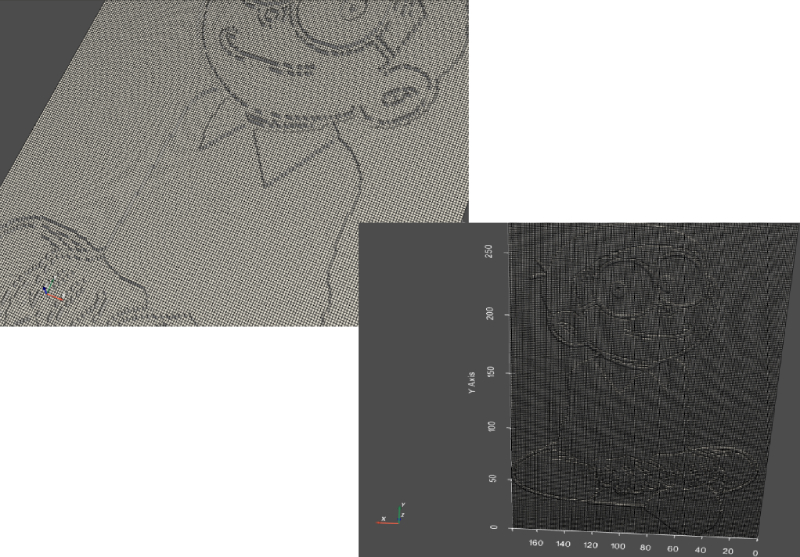• +91-9872993883
• +91-8283824812
• info@ris-ai.com

# Create 3D Model of Photo¶

In this article, we will review some of the functions to create a 3D Model of photo . In the first lines, we import the necessary libraries then read the target image from folder.

In :
import numpy as np
import matplotlib.pyplot as plt
from mpl_toolkits.mplot3d import Axes3D
import scipy.ndimage as ndimage

# path of an image

# get the first channel
mat = mat[:,:,0]
rows, cols = mat.shape

# numpy.meshgrid returns the coordinate matrices from the coordinate vectors
xv, yv = np.meshgrid(range(cols), range(rows)[::-1])

# blur an image using Gaussian filter
blurred = ndimage.gaussian_filter(mat, sigma=(5, 5), order=0)
fig = plt.figure(figsize=(10,10))

ax.imshow(mat, cmap='gray')

# 3d axes are created by passing the projection=3d

# elevation is set to 90
ax.elev= 90

# plot surface
ax.plot_surface(xv, yv, mat)

# show blur image in gray color
ax.imshow(blurred, cmap='gray')
ax.elev= 278
ax.plot_surface(xv, yv, blurred)
plt.show()


### view imageIn :
mat.shape

Out:
(281, 179)

### PyVista¶

PyVista is a supporting module for VTK. It has a Visualization Toolkit (VTK) which is used for wrapping the visualization toolkit library by using numpy. 2D pyvista.StructuredGrid can be extended into 3D mesh.

To install:- pip install pyvista

In :
import pyvista as pv

# pyvista.StructureGrid(x,y,z)
surface = pv.StructuredGrid(xv, yv, mat)

# Plot surface
surface.plot(show_edges=True, show_grid=True, notebook=False)

# save surface
surface.save("my_surface.vtk")